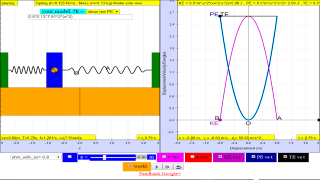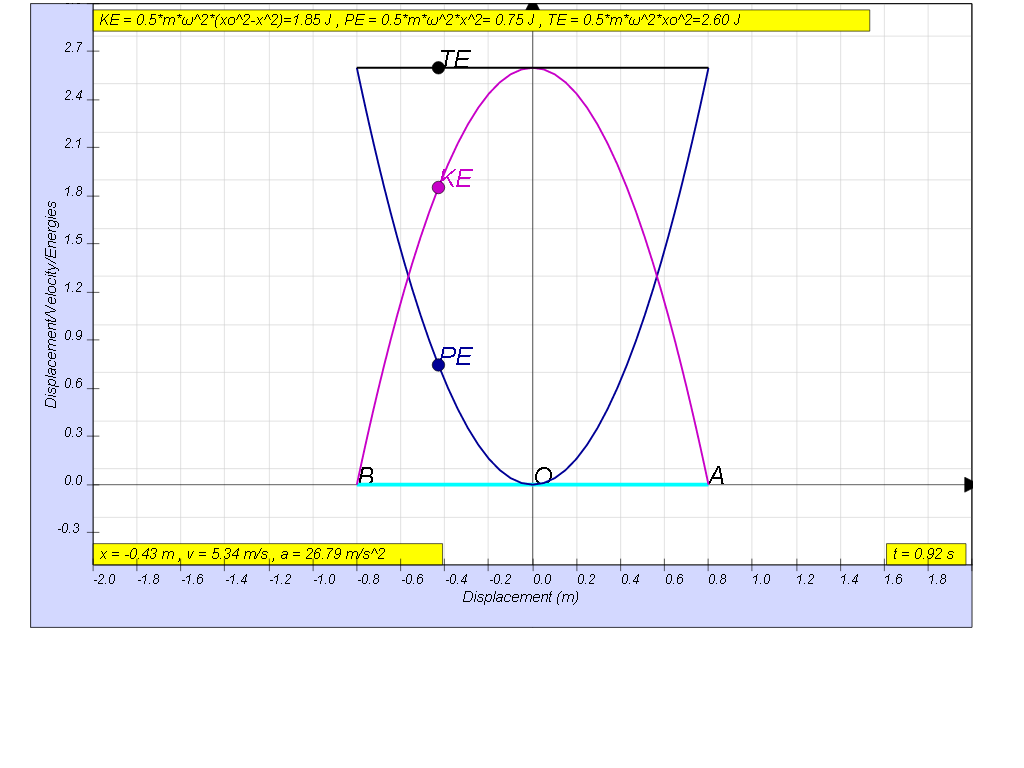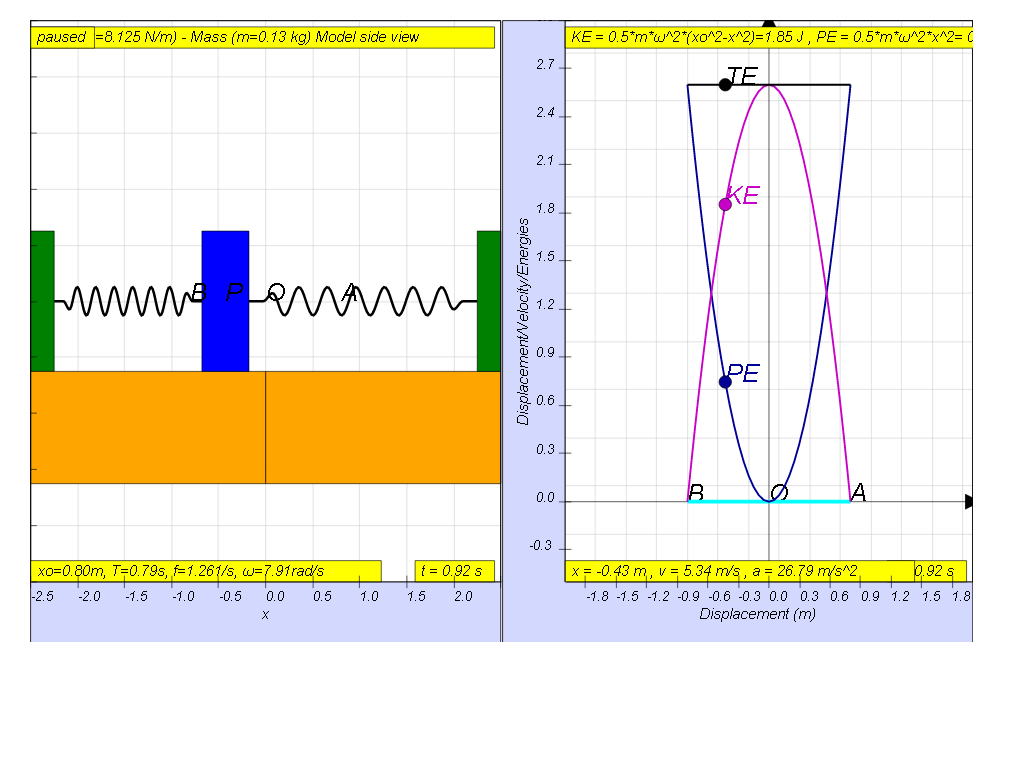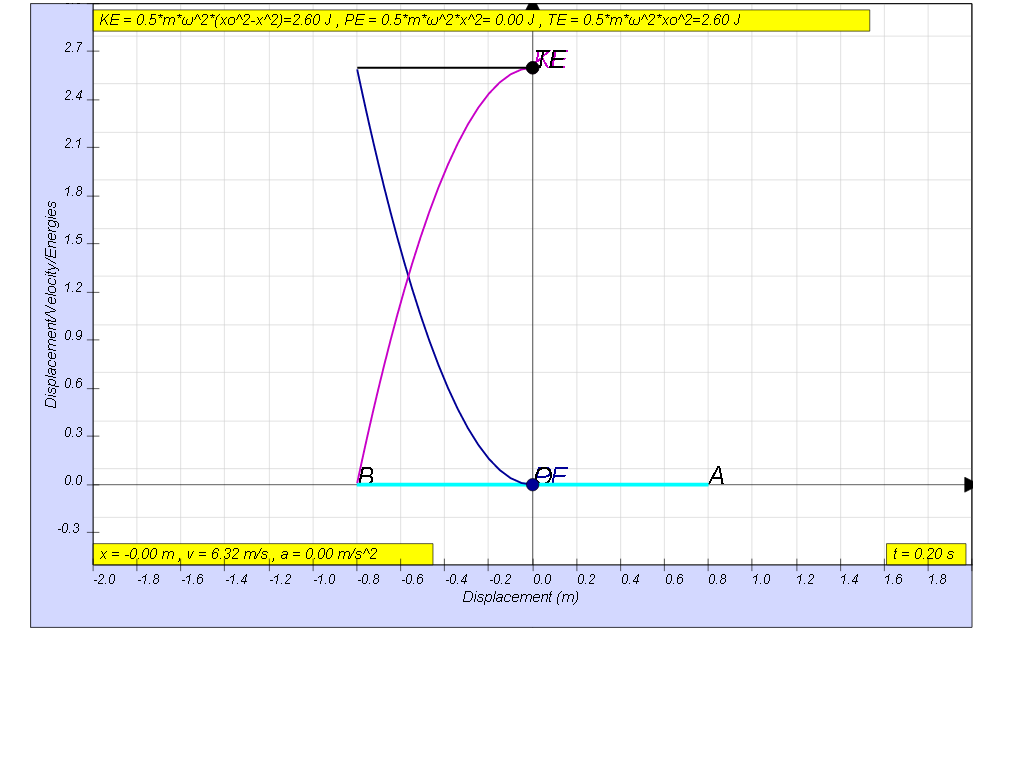SHM19

1.4.3 Example

The mass is displaced to the left from its equilibrium position through a small distance d and is released. The mass undergoes simple harmonic motion.
The graph shows the variation with displacement x from the equilibrium position of the kinetic energy of the mass.Use the graph to
(a)     determine the distance d and the greatest acceleration through which the mass was displaced initially,
(b)    determine the period, frequency and angular frequency.

(c) determine the corresponding equations of displacement, velocity and acceleration.

(d) determine the corresponding equations of elastic potential energy and the total mechanical energy and sketch them on the graph above.

(e) determine the corresponding equations of kinetic energy, potential energy and total energy

[0.8m, 0.79 s, 1.26 Hz, 7.91 rad/s]

[ x= - 0.8 cos(7.91t), v= 6.32 sin(7.91t), a= 50 cos(7.91t)]

[KE = 2.6 sin 7.912t, PE = 2.6 cos 7.912t, TE =2.6 J]

[KE = 4.06 (0.82-x2), PE = 4.06 x2, TE = 2.6 J]

Using the model, this graph can be shownthe velocity maximum value can be found in the model as well1.4.3.1 Model:

Translations

Code Language Translator RunSoftware Requirements

SoftwareRequirements

 Android iOS Windows MacOS with best with Chrome Chrome Chrome Chrome support full-screen? Yes. Chrome/Opera No. Firefox/ Samsung Internet Not yet Yes Yes cannot work on some mobile browser that don't understand JavaScript such as..... cannot work on Internet Explorer 9 and below

CreditsThis email address is being protected from spambots. You need JavaScript enabled to view it.

end faq

http://iwant2study.org/lookangejss/02_newtonianmechanics_8oscillations/ejss_model_SHM19/SHM19_Simulation.xhtml

Testimonials (0)

There are no testimonials available for viewing. Login to deploy the article and be the first to submit your review!